Tamilnadu State Board New Syllabus Samacheer Kalvi 12th Business Maths Guide Pdf Chapter 1 Applications of Matrices and Determinants Ex 1.4 Text Book Back Questions and Answers, Notes.

## Tamilnadu Samacheer Kalvi 12th Business Maths Solutions Chapter 1 Applications of Matrices and Determinants Ex 1.4

Choose the most suitable answer from the given four alternatives:

Question 1.
A = (1, 2, 3), then the rank of AAT is
(a) 0
(b) 2
(c) 3
(d) 1
Solution:
(d) 1
Hint:
A = (1, 2, 3) then
AT = $$\left(\begin{array}{l} 1 \\ 2 \\ 3 \end{array}\right)$$
AAT = (1, 2, 3) $$\left(\begin{array}{l} 1 \\ 2 \\ 3 \end{array}\right)$$ = (1 + 4 + 9) = (14)
Number of non-zero rows = 1
∴ P (AAT) = 1Question 2.
The rank of m × n matrix whose elements are unity is
(a) 0
(b) 1
(c) m
(d) n
Solution:
(b) 1

Question 3.
If T =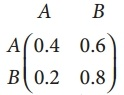is a transition probability matrix, then at equilibriuium A is equal to
(a) $$\frac { 1 }{ 4 }$$
(b) $$\frac { 1 }{ 5 }$$
(c) $$\frac { 1 }{ 6 }$$
(d) $$\frac { 1 }{ 8 }$$
Solution:
(a) $$\frac { 1 }{ 4 }$$
Hint:At equilibrium (A B) $$\left(\begin{array}{ll} 0.4 & 0.6 \\ 0.2 & 0.8 \end{array}\right)$$
0.4 A + 0.2 B = A
0.4 A + 0.2(1 – A) = A
0.4 A + 0.2 – 0.2 A = A
0.2 A + 0.2 = A
0.2 = A – 0.2 A
0.2 = 0.8 A
A = $$\frac { 0.2 }{ 0.8 }$$ = $$\frac { 1 }{ 4 }$$

Question 4.
If A = $$\left(\begin{array}{ll} 2 & 0 \\ 0 & 8 \end{array}\right)$$, then p(A) is
(a) 0
(b) 1
(c) 2
(d) n
Solution:
(c) 2
Hint:
A = $$\left(\begin{array}{ll} 2 & 0 \\ 0 & 8 \end{array}\right)$$
No. of Non-zero rows = 2
∴ p(A) = 2Question 5.
The rank of the matrix $$\left[\begin{array}{lll} 1 & 1 & 1 \\ 1 & 2 & 3 \\ 1 & 4 & 9 \end{array}\right]$$ is
(a) 0
(b) 1
(c) 2
(d) 3
Solution:
(d) 3
Hint: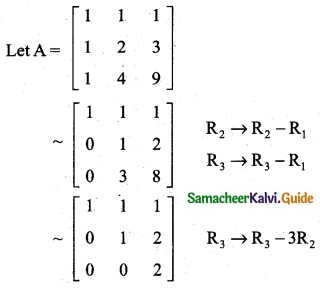No. of Non-zero rows = 3
∴ p(A) = 3

Question 6.
The rank of the unit matrix of order n is
(a) n – 1
(b) n
(c) n + 1
(d) n²
Solution:
(b) n

Question 7.
If p(A) = r then which of the following is correct?
(a) all the minors of order r which does not vanish
(b) A has at least one minor of order r which does not vanish
(c) A has at least one (r + 1) order minor which vanishes
(d) all (r + 1) and higher order minors should not vanish
Solution:
(b) A has at least one minor of order r which does not vanish.Question 8.
If A = $$\left(\begin{array}{l} 1 \\ 2 \\ 3 \end{array}\right)$$ then the rank of AAT is
(a) 0
(b) 1
(c) 2
(d) 3
Solution:
(b) 1
Hint: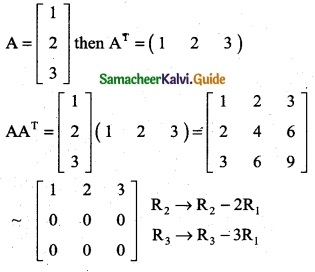No. of non-zero rows = 1
p (AAT) = 1

Question 9.
If the rank of the matrix $$\left[\begin{array}{ccc} \lambda & -1 & 0 \\ 0 & \lambda & -1 \\ -1 & 0 & \lambda \end{array}\right]$$ is 2. then λ is
(a) 1
(b) 2
(c) 3
(d) only real number
Solution:
(a) 1
Hint:
A = $$\left[\begin{array}{ccc} \lambda & -1 & 0 \\ 0 & \lambda & -1 \\ -1 & 0 & \lambda \end{array}\right]$$
Since the Rank is 2, third order matrix vanishes
∴ |A| = 0
$$\left[\begin{array}{ccc} \lambda & -1 & 0 \\ 0 & \lambda & -1 \\ -1 & 0 & \lambda \end{array}\right]$$ = 0
λ(λ² – 0) + 1 (0 – 1) = 0
λ³ – 1 = 0 ⇒ λ³ = 1
∴ λ = 1

Question 10.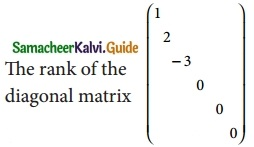(a) 0
(b) 2
(c) 3
(d) 5
Solution:
(c) 3
Hint:
Number of non-zero rows = 3
∴ Rank = 3Question 11.
Ifis a transition probability matrix, then the value of x is
(a) 0.2
(b) 0.3
(c) 0.4
(d) 0.7
Solution:
(c) 0.4
Hint: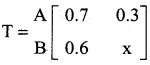is a transition probability matrix then 0.6 + x = 1
x = 1 – 0.6
x = 0.4

Question 12.
Which of the following is not an elementary transformation?
(a) Ri ↔ Rj
(b) Ri → 2Ri + 2Cj
(c) Ri → 2Ri + 4Rj
(d) Ci → Ci + 5Cj
Solution:
(b) Ri → 2Ri + 2Cj

Question 13.
If p(A) = p(A,B)= then the system is
(a) Consistent and has infinitely many solutions
(b) Consistent and has a unique solution
(c) inconsistent
(d) consistent
Solution:
(d) ConsistentQuestion 14.
If p(A) = p(A,B)= the number of unknowns, then the system is
(a) Consistent and has infinitely many solutions
(b) Consistent and has a unique solution
(c) inconsistent
(d) consistent
Solution:
(b) Consistent and has a unique solution

Question 15.
If p(A) ≠ p(A, B) =, then the system is
(a) Consistent and has infinitely many solutions
(b) Consistent and has a unique solution
(c) inconsistent
(d) consistent
Solution:
(c) inconsistent

Question 16.
In a transition probability matrix, all the entries are greater than or equal to
(a) 2
(b) 1
(c) 0
(d) 3
Solution:
(c) 0Question 17.
If the number of variables in a non-homogeneous system AX = B is n, then the system possesses a unique solution only when
(a) p(A) = p(A, B) > n
(b) p(A) = p(A, B) = n
(c) p(A) = p(A, B) < n
(d) none of these
Solution:
(b) p(A) = p(A, B) = n

Question 18.
The system of equations 4x + 6y = 5, 6x + 9y = 7 has
(a) a unique solution
(b) no solution
(c) infinitely many solutions
(d) none of these
Solution:
(b) no solution
Hint:
The matrix form of the equations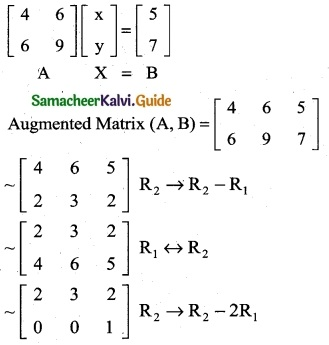p(A) ≠ p(A, B)
∴ The system is inconsistent.

Question 19.
For the system of equations x + 2y + 3z = 1, 2x + y – z = 3, 3x + 2y + k = 4
(a) there is only one solution
(b) there exists infinitely many solution
(c) there is no solution
(d) none of these
Solution:
(a) there is only one solution
Hint:
The matrix form of the equationsThe last equivalent matrix in the echelon form
p(A) = p (A, B) = no. of unknowns
∴ The system is consistent.Question 20.
If |A| ≠ 0, then A is
(a) non – singular matrix
(b) singular matrix
(c) zero matrix
(d) none of these
Solution:
(a) non-singular matrix

Question 21.
The system of linear equations x = y + z = 2, 2x + y – z = 3, 3x + 2y + k = 4 has unique solution, if k is not equal to
(a) 1
(b) 0
(c) 3
(d) 7
Solution:
(b) 0
Hint:
The matrix form of the equations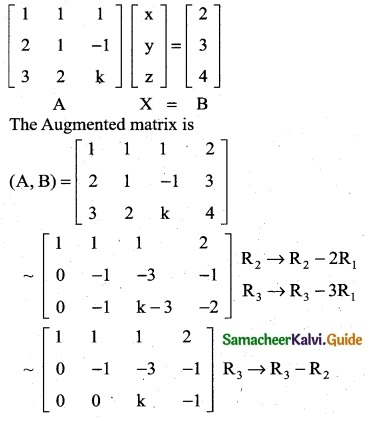Since the system has unique solution.
P(A) = p(A, B) ≠ n
∴ K ≠ 0

Question 22.
Cramer’s rule is applicable only to get an unique solution when
(a) Δz ≠ 0
(b) Δx ≠ 0
(c) Δ ≠ 0
(d) Δy ≠ 0
Solution:
(c) Δ ≠ 0Question 23.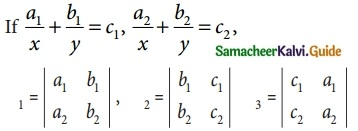Then (x, y) is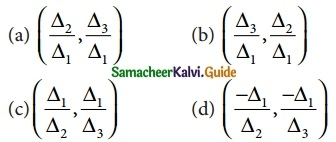Solution:
(d) ($$\frac { -Δ_1 }{ Δ_2 }$$, $$\frac { -Δ_1 }{ Δ_3 }$$)

Question 24.
|An × n| = 3 |adj A| = 243 = (3)5 then the value n is
(a) 4
(b) 5
(c) 6
(d) 7
Solution:
(c) 6

Question 25.
Rank of a null matrix is
(a) 0
(b) -1
(c) ∞
(d) 1
Solution:
(a) 0